original default score was .11543, new best score is .11356

# Stacked and Ensembled Regressions to predict House Prices¶

## Donald S¶

June 2020

Need to submit every 2 months as the leaderboard will rollover after this 2 month period

Typical flow of model building: Use GridSearch for determining Best parameters => **bestestimator

good basic resource for models: https://scikit-learn.org/stable/modules/cross_validation.html https://scikit-learn.org/stable/modules/grid_search.html

A search consists of: an estimator (regressor or classifier such as sklearn.svm.SVC());
a parameter space;
a method for searching or sampling candidates;
a cross-validation scheme; and
a score function. **

estimator.get_params() # get all hyperparameters

inner_cv = KFold(n_splits=4, shuffle=True, random_state=None)

# To be used in outer CV
outer_cv = KFold(n_splits=4, shuffle=True, random_state=None)

#inner loop KFold example:
gsc = GridSearchCV(
estimator=rf,
param_grid=param_grid,
#scoring='roc_auc', # or 'r2', etc
scoring='f1',
#scoring='neg_mean_squared_error', # or look here for other choices
# https://scikit-learn.org/stable/modules/model_evaluation.html#scoring-parameter
#cv=5,
cv=inner_cv, # this will use KFold splitting to change train/test/validation datasets randomly
verbose=verbose
)

print("Running GridSearchCV:")
with MyTimer():
grid_result = gsc.fit(X_train, y_train)
non_nested_scores[i] = grid_result.best_score_

rf = RandomForestClassifier(**grid_result.best_params_)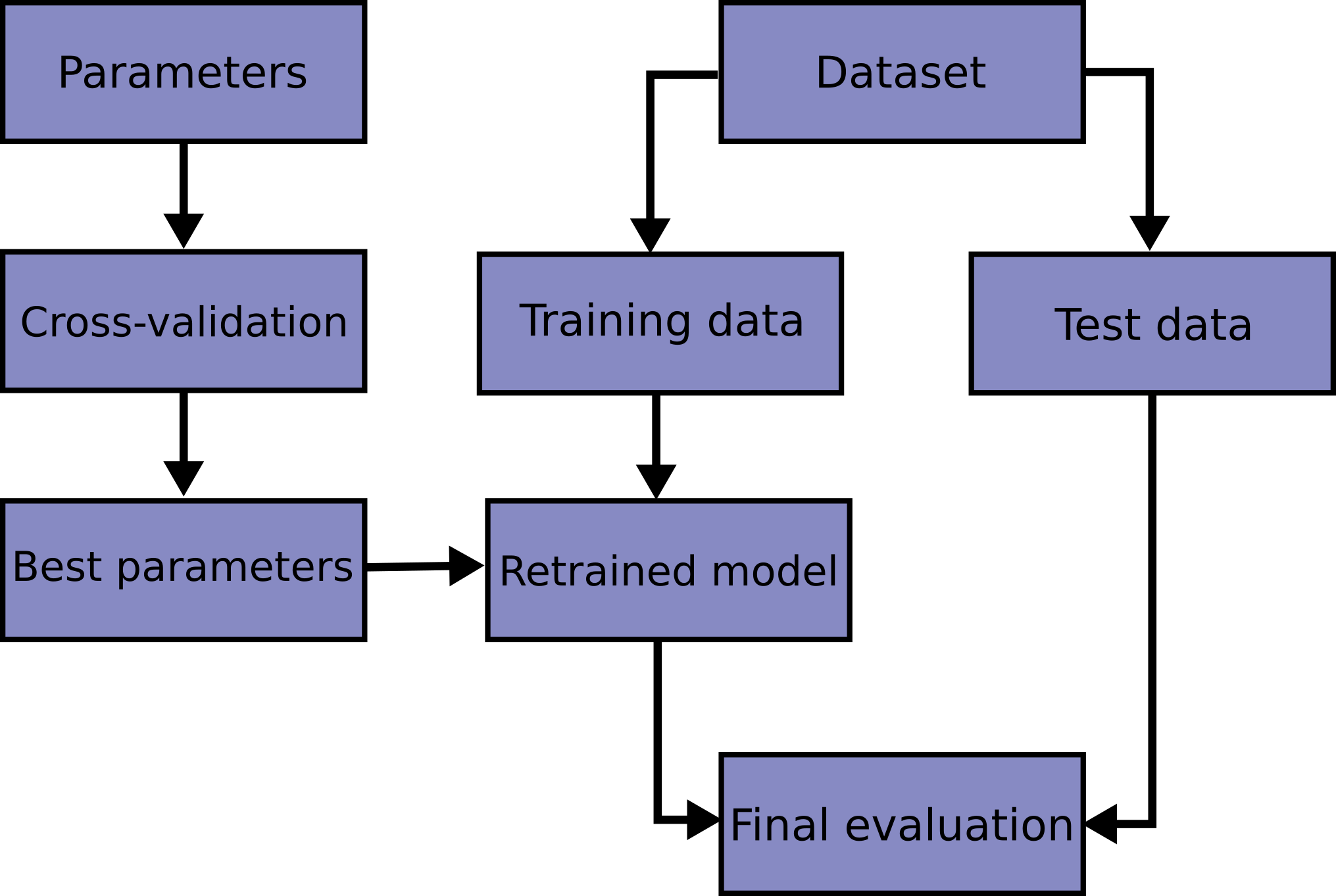This competition is very important to me as it helped me to begin my journey on Kaggle few months ago. I've read some great notebooks here. To name a few:

1. Comprehensive data exploration with Python by Pedro Marcelino : Great and very motivational data analysis

2. A study on Regression applied to the Ames dataset by Julien Cohen-Solal : Thorough features engeneering and deep dive into linear regression analysis but really easy to follow for beginners.

3. Regularized Linear Models by Alexandru Papiu : Great Starter kernel on modelling and Cross-validation

I can't recommend enough every beginner to go carefully through these kernels (and of course through many others great kernels) and get their first insights in data science and kaggle competitions.

After that (and some basic pratices) you should be more confident to go through this great script by Human Analog who did an impressive work on features engeneering.

As the dataset is particularly handy, I decided few days ago to get back in this competition and apply things I learnt so far, especially stacking models. For that purpose, we build two stacking classes ( the simplest approach and a less simple one).

As these classes are written for general purpose, you can easily adapt them and/or extend them for your regression problems. The overall approach is hopefully concise and easy to follow..

The features engeneering is rather parsimonious (at least compared to some others great scripts) . It is pretty much :

• Imputing missing values by proceeding sequentially through the data

• Transforming some numerical variables that seem really categorical

• Label Encoding some categorical variables that may contain information in their ordering set

• Box Cox Transformation of skewed features (instead of log-transformation) : This gave me a slightly better result both on leaderboard and cross-validation.

• Getting dummy variables for categorical features.

Then we choose many base models (mostly sklearn based models + sklearn API of DMLC's XGBoost and Microsoft's LightGBM), cross-validate them on the data before stacking/ensembling them. The key here is to make the (linear) models robust to outliers. This improved the result both on LB and cross-validation.

To my surprise, this does well on LB ( 0.11420 and top 4% the last time I tested it : July 2, 2017 )

Hope that at the end of this notebook, stacking will be clear for those, like myself, who found the concept not so easy to grasp

In :
#import some necessary libraries

import numpy as np # linear algebra
import pandas as pd # data processing, CSV file I/O (e.g. pd.read_csv)
%matplotlib inline
import matplotlib.pyplot as plt  # Matlab-style plotting
import seaborn as sns
color = sns.color_palette()
sns.set_style('darkgrid')
import warnings
def ignore_warn(*args, **kwargs):
pass
warnings.warn = ignore_warn #ignore annoying warning (from sklearn and seaborn)

from scipy import stats
from scipy.stats import norm, skew, kurtosis, boxcox #for some statistics
from scipy.special import boxcox1p, inv_boxcox

pd.set_option('display.float_format', lambda x: '{:.3f}'.format(x)) #Limiting floats output to 3 decimal points

from subprocess import check_output
print(check_output(["ls", "../input"]).decode("utf8")) #check the files available in the directory

data_description.txt
sample_submission.csv
test.csv
train.csv


In :
#Now let's import and put the train and test datasets in  pandas dataframe


In :
##display the first five rows of the train dataset.

Out:
Id MSSubClass MSZoning LotFrontage LotArea Street Alley LotShape LandContour Utilities ... PoolArea PoolQC Fence MiscFeature MiscVal MoSold YrSold SaleType SaleCondition SalePrice
0 1 60 RL 65.000 8450 Pave NaN Reg Lvl AllPub ... 0 NaN NaN NaN 0 2 2008 WD Normal 208500
1 2 20 RL 80.000 9600 Pave NaN Reg Lvl AllPub ... 0 NaN NaN NaN 0 5 2007 WD Normal 181500
2 3 60 RL 68.000 11250 Pave NaN IR1 Lvl AllPub ... 0 NaN NaN NaN 0 9 2008 WD Normal 223500
3 4 70 RL 60.000 9550 Pave NaN IR1 Lvl AllPub ... 0 NaN NaN NaN 0 2 2006 WD Abnorml 140000
4 5 60 RL 84.000 14260 Pave NaN IR1 Lvl AllPub ... 0 NaN NaN NaN 0 12 2008 WD Normal 250000

5 rows × 81 columns

In :
##display the first five rows of the test dataset.

Out:
Id MSSubClass MSZoning LotFrontage LotArea Street Alley LotShape LandContour Utilities ... ScreenPorch PoolArea PoolQC Fence MiscFeature MiscVal MoSold YrSold SaleType SaleCondition
0 1461 20 RH 80.000 11622 Pave NaN Reg Lvl AllPub ... 120 0 NaN MnPrv NaN 0 6 2010 WD Normal
1 1462 20 RL 81.000 14267 Pave NaN IR1 Lvl AllPub ... 0 0 NaN NaN Gar2 12500 6 2010 WD Normal
2 1463 60 RL 74.000 13830 Pave NaN IR1 Lvl AllPub ... 0 0 NaN MnPrv NaN 0 3 2010 WD Normal
3 1464 60 RL 78.000 9978 Pave NaN IR1 Lvl AllPub ... 0 0 NaN NaN NaN 0 6 2010 WD Normal
4 1465 120 RL 43.000 5005 Pave NaN IR1 HLS AllPub ... 144 0 NaN NaN NaN 0 1 2010 WD Normal

5 rows × 80 columns

In :
#check the numbers of samples and features
print("The train data size before dropping Id feature is : {} ".format(train.shape))
print("The test data size before dropping Id feature is : {} ".format(test.shape))

#Save the 'Id' column
train_ID = train['Id']
test_ID = test['Id']

#Now drop the  'Id' colum since it's unnecessary for  the prediction process.
train.drop("Id", axis = 1, inplace = True)
test.drop("Id", axis = 1, inplace = True)

#check again the data size after dropping the 'Id' variable
print("\nThe train data size after dropping Id feature is : {} ".format(train.shape))
print("The test data size after dropping Id feature is : {} ".format(test.shape))

The train data size before dropping Id feature is : (1460, 81)
The test data size before dropping Id feature is : (1459, 80)

The train data size after dropping Id feature is : (1460, 80)
The test data size after dropping Id feature is : (1459, 79)


# Data Processing¶

## Outliers¶

Documentation for the Ames Housing Data indicates that there are outliers present in the training data

Let's explore these outliers

In :
fig, ax = plt.subplots()
ax.scatter(x = train['GrLivArea'], y = train['SalePrice'])
plt.ylabel('SalePrice', fontsize=13)
plt.xlabel('GrLivArea', fontsize=13)

m, b = np.polyfit(train['GrLivArea'], train['SalePrice'], 1)
#m = slope, b=intercept
plt.plot(train['GrLivArea'], m*train['GrLivArea'] + b)

plt.show()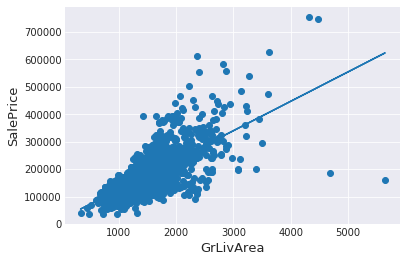Edit: Look for fliers in other columns

In :
train.shape
#a = int(np.sqrt(train.shape))
a = 4
b = int(train.shape/4)
r = int(train.shape/a)
c = int(train.shape/b)
i = 0
fig, ax = plt.subplots(nrows=r, ncols=c, figsize=(15, 60))
for row in ax:
for col in row:
#print(train.columns[i])
#print(train[train.columns[i]].dtype)
#col.plot()
#ax.scatter(x = train['GrLivArea'], y = train['SalePrice'])
try:
col.scatter(x = train[train.columns[i]], y = train['SalePrice'])
col.title.set_text(train.columns[i])
#col.title(train.columns[i])
except:
temp=1
#except Exception as e:
#    print(e.message, e.args)
finally:
temp=1
i = i + 1

plt.show()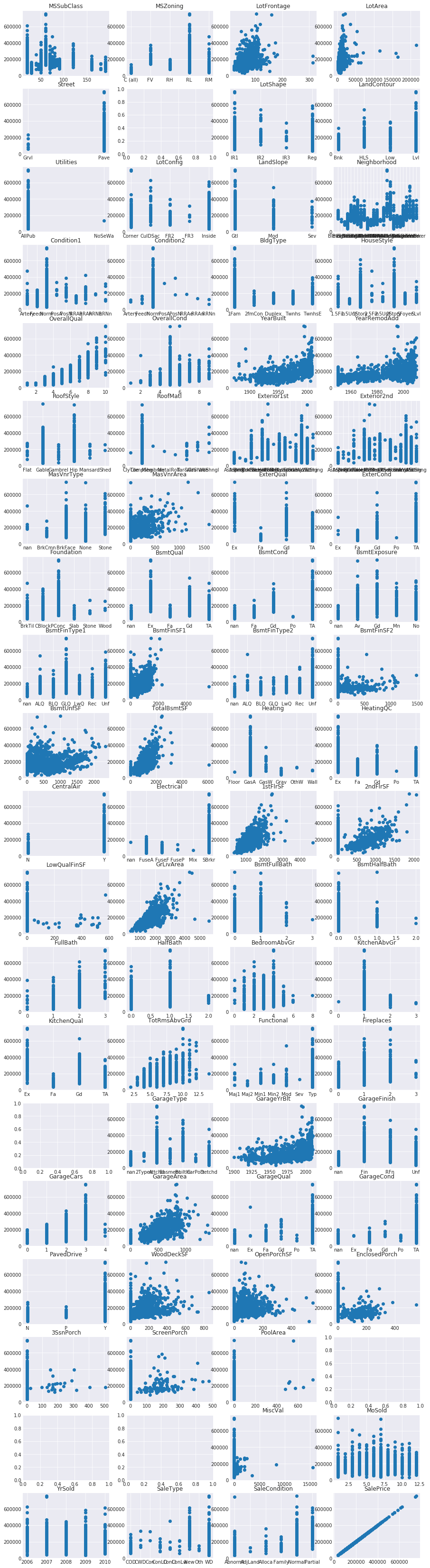More filtering of data to try

In :
#train = train.drop(train[(train['LotFrontage']>300) & (train['SalePrice']<400000)].index)
#train = train.drop(train[(train['LotArea']>100000) & (train['SalePrice']<400000)].index)
#train = train.drop(train[(train['BsmtFinSF1']>4000) & (train['SalePrice']<250000)].index)
#train = train.drop(train[(train['GrLivArea']>4000) & (train['SalePrice']<250000)].index)


We can see at the bottom right two with extremely large GrLivArea that are of a low price. These values are huge oultliers. Therefore, we can safely delete them.

Edit: Think this deletion is safe to do as there are only 2 points and they seem to be abnormal, possibly data error

In :
#Deleting outliers
train = train.drop(train[(train['GrLivArea']>4000) & (train['SalePrice']<300000)].index)

#Check the graphic again
fig, ax = plt.subplots()
ax.scatter(train['GrLivArea'], train['SalePrice'])
plt.ylabel('SalePrice', fontsize=13)
plt.xlabel('GrLivArea', fontsize=13)
m, b = np.polyfit(train['GrLivArea'], train['SalePrice'], 1)
#m = slope, b=intercept
plt.plot(train['GrLivArea'], m*train['GrLivArea'] + b)
plt.show()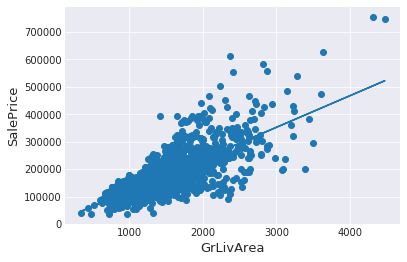### Note :¶

Outliers removal is note always safe. We decided to delete these two as they are very huge and really bad ( extremely large areas for very low prices).

There are probably others outliers in the training data. However, removing all them may affect badly our models if ever there were also outliers in the test data. That's why , instead of removing them all, we will just manage to make some of our models robust on them. You can refer to the modelling part of this notebook for that.

Edit: todo - look for other outliers in all columns. Maybe better to use StandardScaler() and np.clip

## Target Variable¶

SalePrice is the variable we need to predict. So let's do some analysis on this variable first.

In :
sns.distplot(train['SalePrice'] , fit=norm)

_skew = skew(train['SalePrice'])
_kurtosis = kurtosis(train['SalePrice'])

# Get the fitted parameters used by the function
(mu, sigma) = norm.fit(train['SalePrice'])
print( '\n mu = {:.2f} and sigma = {:.2f}, skew = {:.2f} kurtosis = {:.2f}\n'.format(mu, sigma, _skew, _kurtosis))

#Now plot the distribution
plt.legend(['Normal dist. ($\mu=$ {:.2f} and $\sigma=$ {:.2f} )'.format(mu, sigma)],
loc='best')
plt.ylabel('Frequency')
plt.title('SalePrice distribution')

#Get also the QQ-plot
fig = plt.figure()
res = stats.probplot(train['SalePrice'], plot=plt)
plt.show()

 mu = 180932.92 and sigma = 79467.79, skew = 1.88 kurtosis = 6.50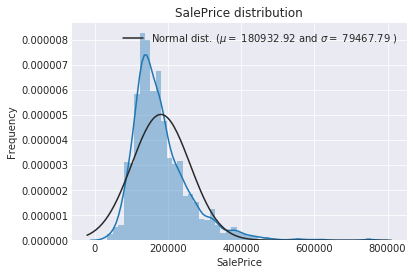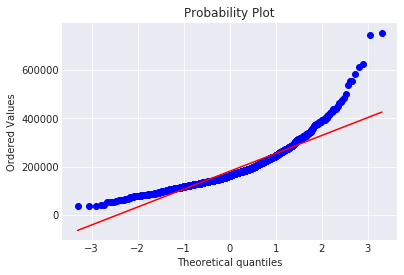The target variable is right skewed. As (linear) models love normally distributed data , we need to transform this variable and make it more normally distributed.

Log-transformation of the target variable

Edit: also trying box-cox transform. The Box-Cox transform is given by:

y = (x**lmbda - 1) / lmbda,  for lmbda > 0
log(x),                  for lmbda = 0


In :
#We use the numpy fuction log1p which  applies log(1+x) to all elements of the column

# option 1
#train["SalePrice"] = np.log1p(train["SalePrice"])

# Option 2: use box-cox transform - this performs worse than the log(1+x), reverting back
lam_l = 0.35
train["SalePrice"] = boxcox1p(train["SalePrice"], lam_l)

# Option 3: boxcox letting the algorithm select lmbda based on least-likelihood calculation
#train["SalePrice"], lam_l = boxcox(x=train["SalePrice"], lmbda=None)

In :
# to revert back use y = inv_boxcox(x, lam_l) => train["SalePrice"] = inv_boxcox(train["SalePrice"], lam_l)
# think the formula is this: # pred_y = np.power((y_box * lambda_) + 1, 1 / lambda_) - 1

#Check the new distribution
sns.distplot(train['SalePrice'] , fit=norm);

_skew = skew(train['SalePrice'])
_kurtosis = kurtosis(train['SalePrice'])

# Get the fitted parameters used by the function
(mu, sigma) = norm.fit(train['SalePrice'])
print( '\n mu = {:.2f} and sigma = {:.2f}, skew = {:.2f} kurtosis = {:.2f}\n'.format(mu, sigma, _skew, _kurtosis))

#Now plot the distribution
plt.legend(['Normal dist. ($\mu=$ {:.2f} and $\sigma=$ {:.2f} )'.format(mu, sigma)],
loc='best')
plt.ylabel('Frequency')
plt.title('SalePrice distribution')

#Get also the QQ-plot
fig = plt.figure()
res = stats.probplot(train['SalePrice'], plot=plt)
plt.show()

 mu = 191.18 and sigma = 27.62, skew = 0.69 kurtosis = 1.31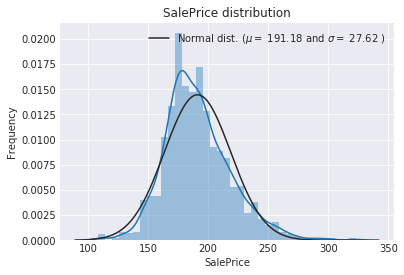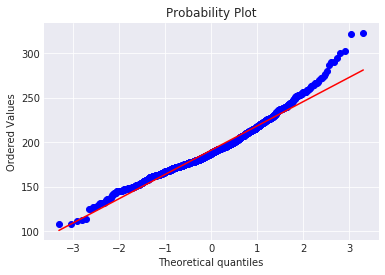The skew seems now corrected and the data appears more normally distributed.

Edit: Both distributions have a positive kurtosis, which means there is a steep dropoff in the curve from the center, or the tails have few points. Skewness is close to 0 now, so that metric is closer to a normal distribution

## Features engineering¶

let's first concatenate the train and test data in the same dataframe

In :
ntrain = train.shape
ntest = test.shape
y_train = train.SalePrice.values
all_data = pd.concat((train, test)).reset_index(drop=True)
all_data.drop(['SalePrice'], axis=1, inplace=True)
print("all_data size is : {}".format(all_data.shape))

all_data size is : (2917, 79)

In :
all_data.head()

Out:
1stFlrSF 2ndFlrSF 3SsnPorch Alley BedroomAbvGr BldgType BsmtCond BsmtExposure BsmtFinSF1 BsmtFinSF2 ... SaleType ScreenPorch Street TotRmsAbvGrd TotalBsmtSF Utilities WoodDeckSF YearBuilt YearRemodAdd YrSold
0 856 854 0 NaN 3 1Fam TA No 706.000 0.000 ... WD 0 Pave 8 856.000 AllPub 0 2003 2003 2008
1 1262 0 0 NaN 3 1Fam TA Gd 978.000 0.000 ... WD 0 Pave 6 1262.000 AllPub 298 1976 1976 2007
2 920 866 0 NaN 3 1Fam TA Mn 486.000 0.000 ... WD 0 Pave 6 920.000 AllPub 0 2001 2002 2008
3 961 756 0 NaN 3 1Fam Gd No 216.000 0.000 ... WD 0 Pave 7 756.000 AllPub 0 1915 1970 2006
4 1145 1053 0 NaN 4 1Fam TA Av 655.000 0.000 ... WD 0 Pave 9 1145.000 AllPub 192 2000 2000 2008

5 rows × 79 columns

### Missing Data¶

In :
all_data_na = (all_data.isnull().sum() / len(all_data)) * 100
all_data_na = all_data_na.drop(all_data_na[all_data_na == 0].index).sort_values(ascending=False)[:30]
missing_data = pd.DataFrame({'Missing Ratio' :all_data_na})

Out:
Missing Ratio
PoolQC 99.691
MiscFeature 96.400
Alley 93.212
Fence 80.425
FireplaceQu 48.680
LotFrontage 16.661
GarageQual 5.451
GarageCond 5.451
GarageFinish 5.451
GarageYrBlt 5.451
GarageType 5.382
BsmtExposure 2.811
BsmtCond 2.811
BsmtQual 2.777
BsmtFinType2 2.743
BsmtFinType1 2.708
MasVnrType 0.823
MasVnrArea 0.788
MSZoning 0.137
BsmtFullBath 0.069
In :
f, ax = plt.subplots(figsize=(15, 12))
plt.xticks(rotation='90')
sns.barplot(x=all_data_na.index, y=all_data_na)
plt.xlabel('Features', fontsize=15)
plt.ylabel('Percent of missing values', fontsize=15)
plt.title('Percent missing data by feature', fontsize=15)

Out:
Text(0.5,1,'Percent missing data by feature')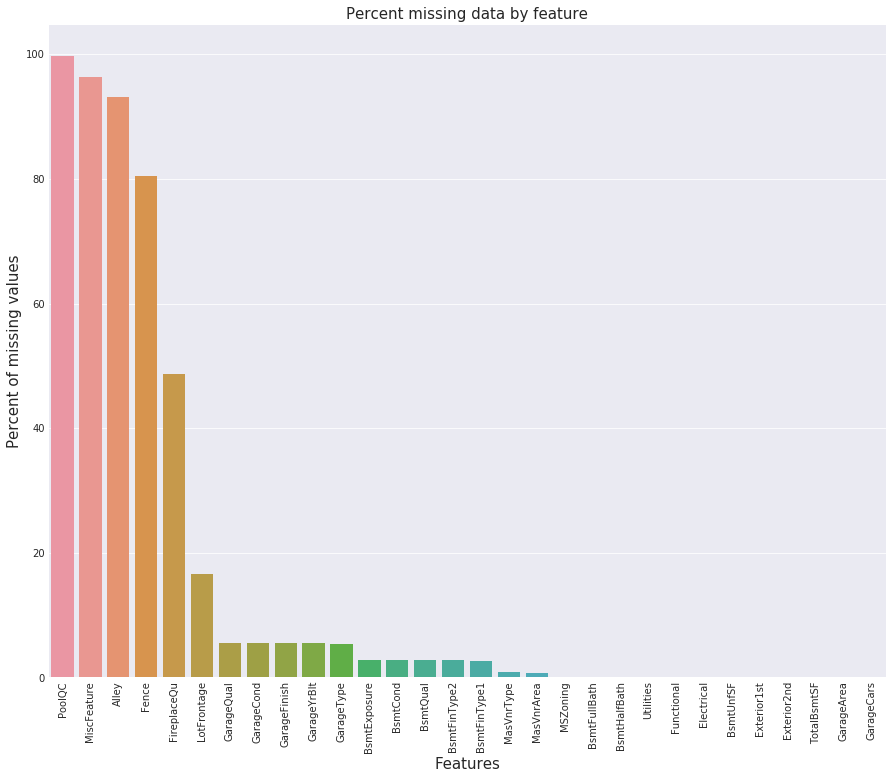Data Correlation

Cross Correlation chart to view collinearity within the features. Kendall's seems appropriate as the Output Variable is numerical and much of the input is categorical. Here is a chart to guide you to which method to use based on your dataset.